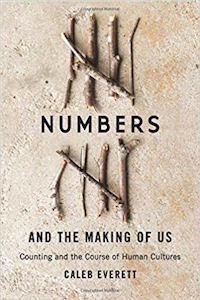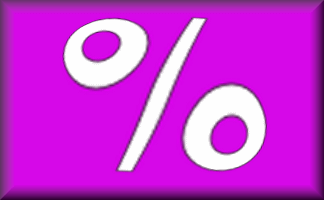# High Interest

 10% of £8.00 = 20% of £8.00 = 30% of £8.00 = 40% of £8.00 = 50% of £8.00 = 60% of £8.00 = 70% of £8.00 = 80% of £8.00 = 90% of £8.00 = 100% of £8.00 = 10% of £8.00 = 5% of £8.00 = 2.5% of £8.00 = 1.25% of £8.00 = 0.625% of £8.00 =Work out the answers to these calculations in your head or on paper.

There are many uses for percentages in real life. Finding a good personal loan for example requires an ability to calculate percentages. Interest is the extra amount you would have to pay back to the personal loan company for borrowing the money. On the other hand you can earn interest by depositing money in a bank or building society.This activity is suitable for students of mathematics all around the world. Use the button below to change the currency symbol used to make it more relevant to your students. You may wish to choose an unfamiliar currency to extend your students' experience.## A Mathematics Lesson Starter Of The Day

Share

Topics: Starter | Percentages

• Fiona Ryan, Moonta Area School
•
• I used this activity as a calculator skills activity for my Special Education class.
• Transum,
•
• This is your chance to get your students to practise the techniques they have learned. This really is a 'skill for life' activity and falls in the financial maths camp too. The ability to mentally calculate simple percentages is so useful but many students find their way through school without learning the tricks and tips. This starter needs discussing with the class when everyone has had a chance to do it. Strategies can be shared, misconceptions challenged and techniques refined.
• Sandra C, London
•
• I used this for a starter on one day, and on the following day, tackled percentages greater than 100% with a look at payday loans.
We also looked at the difference between interest rate p.a. and APR.
• Mrs Boyce,
•
• School starts for us here is just two short weeks, so I am excited to make Transum a part of that school day beyond just the starters! I would like to say one more thing...I truly appreciate you making the little buttons at the bottom of the page that change pounds to dollars and such! It is fun to have the students see and learn the symbol, and talk about how it works, and how it compares to US currency. Then it leads to discussion about money conversion from country to country.

Anyhow, I just wanted to take a moment and say well done! Your site is very well thought out. I appreciate your work very much.

How did you use this starter? Can you suggest how teachers could present or develop this resource? Do you have any comments? It is always useful to receive feedback and helps make this free resource even more useful for Maths teachers anywhere in the world.

If you don't have the time to provide feedback we'd really appreciate it if you could give this page a score! We are constantly improving and adding to these starters so it would be really helpful to know which ones are most useful. Simply click on a button below:

Excellent, I would like to see more like this
Good, achieved the results I required
Satisfactory
Didn't really capture the interest of the students
Not for me! I wouldn't use this type of activity.

This starter has scored a mean of 2.5 out of 5 based on 67 votes.

Previous Day | This starter is for 26 August | Next Day

% - This is the percent symbol.
Percent means 'out of 100'.

### Find 50%:

As 50 is half of 100, then 50% means half. To find 50% of a quantity you need to halve (or divide by two). So 50% of 6 is 3.

### Find 10%:

As 10 is one tenth of 100, then 10% means 'one tenth of'. To find 10% of a quantity you need to divide it by ten. So 10% of 800 is 80.

### Find 25%:

As 25 is one quarter of 100, then 25% means 'one quarter of'. To find 25% of a quantity you need to divide it by four. So 25% of 20 is 5.

Another way of finding 25% of a quantity is first finding 50% then dividing the result by 2.

### Find 33⅓%:

As 33⅓ is one third of 100, then 33⅓% means 'one third of'. To find 33⅓% of a quantity you need to divide it by three. So 33⅓% of 30 is 10.

### Find 1%:

As 1 is one hundredth of 100, then 1% means 'one hundredth of'. To find 1% of a quantity you need to divide it by 100. So 1% of 800 is 8.

### Find other percentages:

Other percentages can be found by combining some of the techniques mentioned above. Here are some examples:

• To find 75% of a quantity add together 50% and 25% of it.
• To find 20% of a quantity double 10% of it.
• To find 5% of a quantity halve 10% of it.
• To find 66⅔% of a quantity double 33⅓% of it.
• To find 90% of a quantity subract 10% from that quantity.
• To find 80% of a quantity subract 20% from that quantity.
• To find 2% of a quantity double 1% of it
• To find 52% of a quantity add 50% to 2% of that quantity

If you need to use a calculator to check your working. See Calculator Workout skill 3.

### Commute

Did you know that if you are struggling to mentally work out 24% of 50 you can switch the numbers round and work out 50% of 24 instead. Finding 50% is very easy isn’t it? You will get the same answer.

Finding a percentage of a quantity is an example of a commutative calculation. Not all operations are commutative. Subtraction certainly isn’t as 10 minus one is not the same as one minus ten.

You can use this trick to improve your ability to do this type of calculation quickly if you find the switch makes it easier.

Practise with 12% of 50, 4% of 25 and 75% of 10.

 10% of £8.00 = £0.80 or 80p 20% of £8.00 = £1.60 30% of £8.00 = £2.40 40% of £8.00 = £3.20 50% of £8.00 = £4.0060% of £8.00 = £4.8070% of £8.00 = £5.6080% of £8.00 = £6.4090% of £8.00 = £7.20100% of £8.00 =£8.00 10% of £8.00 = £0.80 or 80p5% of £8.00 = 40p2.5% of £8.00 = 20p1.25% of £8.00 = 10p0.625% of £8.00 = 5p

Note to teacher: Doing this activity once with a class helps students develop strategies. It is only when they do this activity a second time that they will have the opportunity to practise those strategies. That is when the learning is consolidated. Click the button above to regenerate another version of this starter from random numbers.

Your access to the majority of the Transum resources continues to be free but you can help support the continued growth of the website by doing your Amazon shopping using the links on this page. Below is an Amazon search box and some items chosen and recommended by Transum Mathematics to get you started.## Numbers and the Making of Us

I initially heard this book described on the Grammar Girl podcast and immediately went to find out more about it. I now have it on my Christmas present wish list and am looking forward to receiving a copy (hint!).

"Caleb Everett provides a fascinating account of the development of human numeracy, from innate abilities to the complexities of agricultural and trading societies, all viewed against the general background of human cultural evolution. He successfully draws together insights from linguistics, cognitive psychology, anthropology, and archaeology in a way that is accessible to the general reader as well as to specialists." more...Teacher, do your students have access to computers?Do they have iPads or Laptops in Lessons? Whether your students each have a TabletPC, a Surface or a Mac, this activity lends itself to eLearning (Engaged Learning).Transum.org/go/?Start=August26

Here is a student interactive percentages exercise:

Transum.org/go/?to=PercentagesFor All: